PSoC™ 4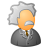Level 1
Level 1

# Advice for Beginner PSoC user required

Hello,

I am entirely new to programming in any language and have purchased a CY8CKIT-042 Pioneer Kit. I have went through PSoC 101 lessons 1-9.

I have some questions from each lesson that may seem trivial but please humour me.

Lesson 1- Question 1

Parallel programming, after the lesson was finished Alan said you should try something new, for me I flashed different LED's, red then green, then blue. I had the idea to make this sequence.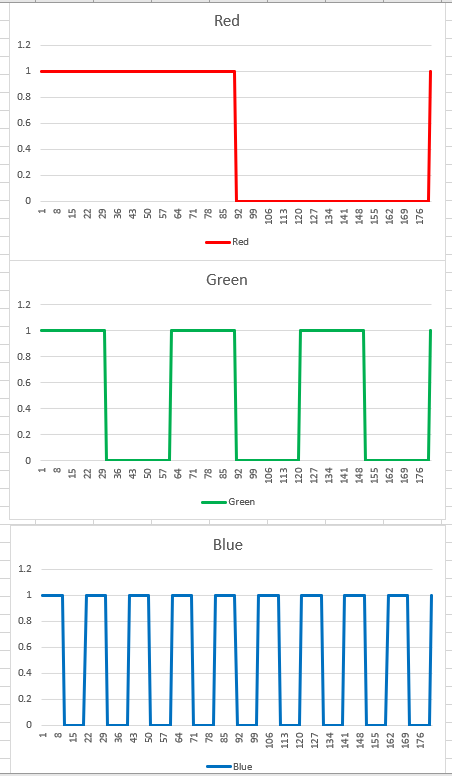Each LED would be flashing on off at a different rate, using the firmware, to do this I would need parallel programming where it would, execute all programs at the same time. I am pretty sure this is possible but cannot find anywhere that shows how it is done. Could you please assist?

1 SolutionLevel 9Level 9

# Re: Advice for Beginner PSoC user required

Hi,

Although you were talking about parallel programming,

my previous samples were quite single-task style.

So today I tried to imitate some parallel programming flavors.

At first I separated doLEDs() function into individual LED handler

do_red(), do_green(), do_blue().

Then I changed the timer to 1ms timer to imitate "tick."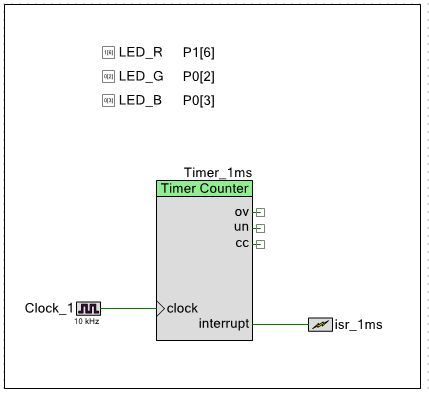And for each "tick", I let each handlers take care of their business.

======================

for(;;) {

if (pit_flag) {

do_red(count) ;

do_green(count) ;

do_blue(count) ;

count = (count + 1) % TIMER_PERIOD ;

pit_flag = 0 ;

}

}

======================

=== led_test_191114A ===

main.c

===============

#include "project.h"

#define LED_ON  (0u)

#define LED_OFF (1u)

#define TIMER_PERIOD 1000

#define RED_PERIOD   1000

#define GREEN_PERIOD  500

#define BLUE_PERIOD   250

volatile int     pit_flag = 0 ;

CY_ISR(timer_isr)

{

pit_flag = 1 ;

}

void do_red(int count)

{

if ((count % RED_PERIOD) < (RED_PERIOD/2)) {

LED_R_Write(LED_OFF) ;

} else {

LED_R_Write(LED_ON) ;

}

}

void do_green(int count)

{

if ((count % GREEN_PERIOD) < (GREEN_PERIOD/2)) {

LED_G_Write(LED_OFF) ;

} else {

LED_G_Write(LED_ON) ;

}

}

void do_blue(int count)

{

if ((count % BLUE_PERIOD) < (BLUE_PERIOD/2)) {

LED_B_Write(LED_OFF) ;

} else {

LED_B_Write(LED_ON) ;

}

}

int main(void)

{

int count = 0 ;

isr_1ms_StartEx(timer_isr) ; /* set isr to the interrupt */

Timer_1ms_Start() ;

CyGlobalIntEnable; /* Enable global interrupts. */

for(;;) {

if (pit_flag) {

do_red(count) ;

do_green(count) ;

do_blue(count) ;

count = (count + 1) % TIMER_PERIOD ;

pit_flag = 0 ;

}

}

}

===============

=== led_test_191114B ===

But with this approach all handlers were called at once,

so I changed the main()  to slice the timing,

so that each task will be called at different tick timing.

Now task(handlers) are called round-robin style.

main loop of led_test_19114B

=======================

for(;;) {

if (pit_flag) {

case 0:

do_red(count) ;

break ;

case 1:

do_green(count) ;

break ;

case 2:

do_blue(count) ;

break ;

default:

break ;

}

count = (count + 1) % TIMER_PERIOD ;

pit_flag = 0 ;

}

}

=======================

=== led_test_191114C ===

Since I came this far,

I introduced a type "task_type" so that main() can call each member of task_type array.

With this, conceptually any numbers of tasks could be handled,

so this can be a very primitive multi task.

main() of led_test_191114C

========================

int main(void)

{

int count = 0 ;

isr_1ms_StartEx(timer_isr) ; /* set isr to the interrupt */

Timer_1ms_Start() ;

CyGlobalIntEnable; /* Enable global interrupts. */

for(;;) {

if (pit_flag) {

count = (count + 1) % TIMER_PERIOD ;

pit_flag = 0 ;

}

}

}

========================

main.c (led_test_191114C)

==========================

#include "project.h"

#define LED_ON  (0u)

#define LED_OFF (1u)

#define TIMER_PERIOD 1000

#define RED_PERIOD   1000

#define GREEN_PERIOD  500

#define BLUE_PERIOD   250

volatile int     pit_flag = 0 ;

CY_ISR(timer_isr)

{

pit_flag = 1 ;

}

void do_red(int count)

{

if ((count % RED_PERIOD) < (RED_PERIOD/2)) {

LED_R_Write(LED_OFF) ;

} else {

LED_R_Write(LED_ON) ;

}

}

void do_green(int count)

{

if ((count % GREEN_PERIOD) < (GREEN_PERIOD/2)) {

LED_G_Write(LED_OFF) ;

} else {

LED_G_Write(LED_ON) ;

}

}

void do_blue(int count)

{

if ((count % BLUE_PERIOD) < (BLUE_PERIOD/2)) {

LED_B_Write(LED_OFF) ;

} else {

LED_B_Write(LED_ON) ;

}

}

int main(void)

{

int count = 0 ;

isr_1ms_StartEx(timer_isr) ; /* set isr to the interrupt */

Timer_1ms_Start() ;

CyGlobalIntEnable; /* Enable global interrupts. */

for(;;) {

if (pit_flag) {

count = (count + 1) % TIMER_PERIOD ;

pit_flag = 0 ;

}

}

}

==========================

If you want to go further, you may want to refer and study Bob-san's master piece,

moto

4 RepliesModeratorModerator

# Re: Advice for Beginner PSoC user required

Hello Stanley,

Thank you for contacting Cypress Technical Support.

I have attached a sample project that implements the sequence mentioned by you.

I have used the three PWM (Pulse Width Modulation) Components which run on the same clock.(1KHz)

I have made the following settings for the three LED:

For Red, period = 4000 counts, compare = 2000 counts

For Green, period = 2000 counts, compare = 1000 counts

For Blue, period = 1000 counts, compare =500.

Thus the duty cycle for each LED remains 50 percent. Also the period Blue led is half of Green led and that of Green led is half of Red led.

Best Regards

EktaLevel 9Level 9

# Re: Advice for Beginner PSoC user required

Hi,

Since this seems to be a fun topic, let me join 😉

Although Ekta-san's gorgeous approach should work fine,

unless you need exact synchronization between 3 signals,

using 3 timers out of 4 available timers sounds a little over kill to me.

So let me post some "poor man's approaches".

At first a very primitive approach of controlling LEDs by software loop, "led_test_191113A"

The schematic is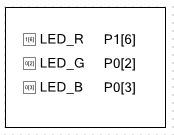main.c

===================

#include "project.h"

#define LED_ON  (0u)

#define LED_OFF (1u)

void doLEDs(uint8_t value)

{

if (value & 0x01) {

LED_B_Write(LED_ON) ;

} else {

LED_B_Write(LED_OFF) ;

}

if (value & 0x02) {

LED_G_Write(LED_ON) ;

} else {

LED_G_Write(LED_OFF) ;

}

if (value & 0x04) {

LED_R_Write(LED_ON) ;

} else {

LED_R_Write(LED_OFF) ;

}

}

int main(void)

{

uint8_t count = 0 ;

CyGlobalIntEnable; /* Enable global interrupts. */

for(;;)

{

doLEDs(count) ;

count = (count + 1) % 8 ; /* count = 0..7 */

CyDelay(1000) ; /* wait 1 sec */

}

}

===================

I think this approach is the most easy to understand,

but as it hogs all the MCU's time, may be not a good idea for real application.

Then I added a timer and interrupt to handle a periodic event, "led_test_191113B"

schematic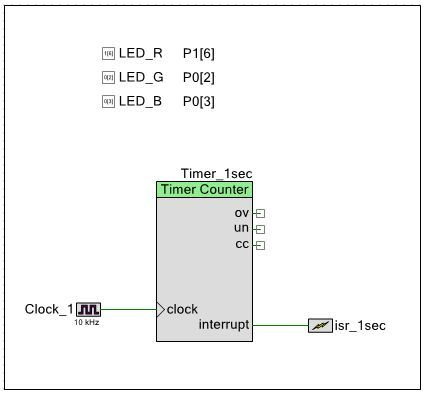main.c

================

#include "project.h"

#define LED_ON  (0u)

#define LED_OFF (1u)

volatile uint8_t count = 0 ;

void doLEDs(uint8_t count) ;

CY_ISR(timer_isr)

{

doLEDs(count) ;

count = (count + 1) % 8 ;

}

void doLEDs(uint8_t value)

{

if (value & 0x01) {

LED_B_Write(LED_ON) ;

} else {

LED_B_Write(LED_OFF) ;

}

if (value & 0x02) {

LED_G_Write(LED_ON) ;

} else {

LED_G_Write(LED_OFF) ;

}

if (value & 0x04) {

LED_R_Write(LED_ON) ;

} else {

LED_R_Write(LED_OFF) ;

}

}

int main(void)

{

isr_1sec_StartEx(timer_isr) ; /* set isr to the interrupt */

Timer_1sec_Start() ;

CyGlobalIntEnable; /* Enable global interrupts. */

for(;;)

{

// MCU is free to do other things.

}

}

================

With this approach it consumes only 1 timer, and most of the MCU power is available for doing other task(s).

If I need exact timing for the 3 signals, probably I will use UDB and make a  3 bit counter "led_test_191113C"

(But this consumes UDB a lot...)

I created an UDB component,

symbol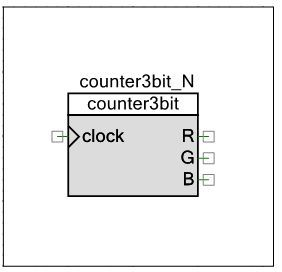verilog source (counter3bit.v)

===============

module counter3bit(

input clock,

output reg R,

output reg G,

output reg B

) ;

reg [15:0] count ;

always @ (posedge clock) begin

{ R, G, B } <= ~count[15:13] ;

count <= count + 3'd1 ;

end

endmodule

===============

schematic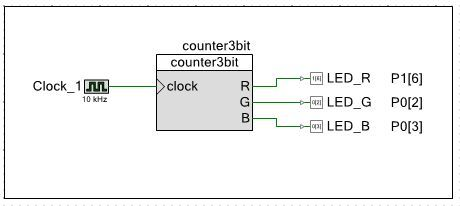main.c

Note: Actually nothing to do from software side...

===========

#include "project.h"

int main(void)

{

CyGlobalIntEnable; /* Enable global interrupts. */

for(;;)

{

}

}

===========

motoLevel 9Level 9

# Re: Advice for Beginner PSoC user required

stem,

The simplest solution would be 3 separate clocks driving output pins. No coding required.

/odissey1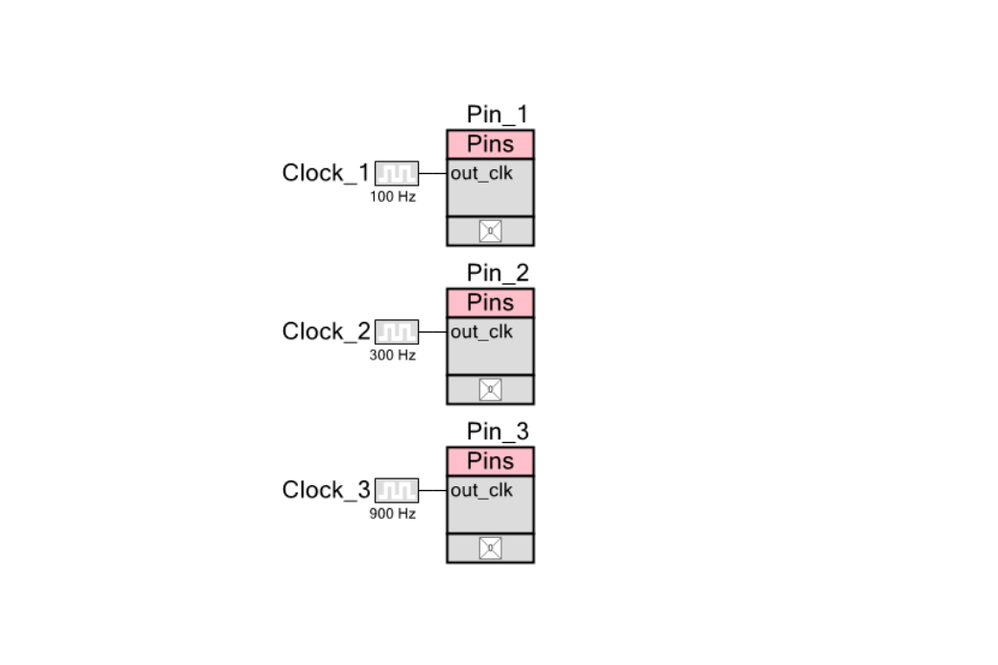Level 9Level 9

# Re: Advice for Beginner PSoC user required

Hi,

Although you were talking about parallel programming,

my previous samples were quite single-task style.

So today I tried to imitate some parallel programming flavors.

At first I separated doLEDs() function into individual LED handler

do_red(), do_green(), do_blue().

Then I changed the timer to 1ms timer to imitate "tick."And for each "tick", I let each handlers take care of their business.

======================

for(;;) {

if (pit_flag) {

do_red(count) ;

do_green(count) ;

do_blue(count) ;

count = (count + 1) % TIMER_PERIOD ;

pit_flag = 0 ;

}

}

======================

=== led_test_191114A ===

main.c

===============

#include "project.h"

#define LED_ON  (0u)

#define LED_OFF (1u)

#define TIMER_PERIOD 1000

#define RED_PERIOD   1000

#define GREEN_PERIOD  500

#define BLUE_PERIOD   250

volatile int     pit_flag = 0 ;

CY_ISR(timer_isr)

{

pit_flag = 1 ;

}

void do_red(int count)

{

if ((count % RED_PERIOD) < (RED_PERIOD/2)) {

LED_R_Write(LED_OFF) ;

} else {

LED_R_Write(LED_ON) ;

}

}

void do_green(int count)

{

if ((count % GREEN_PERIOD) < (GREEN_PERIOD/2)) {

LED_G_Write(LED_OFF) ;

} else {

LED_G_Write(LED_ON) ;

}

}

void do_blue(int count)

{

if ((count % BLUE_PERIOD) < (BLUE_PERIOD/2)) {

LED_B_Write(LED_OFF) ;

} else {

LED_B_Write(LED_ON) ;

}

}

int main(void)

{

int count = 0 ;

isr_1ms_StartEx(timer_isr) ; /* set isr to the interrupt */

Timer_1ms_Start() ;

CyGlobalIntEnable; /* Enable global interrupts. */

for(;;) {

if (pit_flag) {

do_red(count) ;

do_green(count) ;

do_blue(count) ;

count = (count + 1) % TIMER_PERIOD ;

pit_flag = 0 ;

}

}

}

===============

=== led_test_191114B ===

But with this approach all handlers were called at once,

so I changed the main()  to slice the timing,

so that each task will be called at different tick timing.

Now task(handlers) are called round-robin style.

main loop of led_test_19114B

=======================

for(;;) {

if (pit_flag) {

case 0:

do_red(count) ;

break ;

case 1:

do_green(count) ;

break ;

case 2:

do_blue(count) ;

break ;

default:

break ;

}

count = (count + 1) % TIMER_PERIOD ;

pit_flag = 0 ;

}

}

=======================

=== led_test_191114C ===

Since I came this far,

I introduced a type "task_type" so that main() can call each member of task_type array.

With this, conceptually any numbers of tasks could be handled,

so this can be a very primitive multi task.

main() of led_test_191114C

========================

int main(void)

{

int count = 0 ;

isr_1ms_StartEx(timer_isr) ; /* set isr to the interrupt */

Timer_1ms_Start() ;

CyGlobalIntEnable; /* Enable global interrupts. */

for(;;) {

if (pit_flag) {

count = (count + 1) % TIMER_PERIOD ;

pit_flag = 0 ;

}

}

}

========================

main.c (led_test_191114C)

==========================

#include "project.h"

#define LED_ON  (0u)

#define LED_OFF (1u)

#define TIMER_PERIOD 1000

#define RED_PERIOD   1000

#define GREEN_PERIOD  500

#define BLUE_PERIOD   250

volatile int     pit_flag = 0 ;

CY_ISR(timer_isr)

{

pit_flag = 1 ;

}

void do_red(int count)

{

if ((count % RED_PERIOD) < (RED_PERIOD/2)) {

LED_R_Write(LED_OFF) ;

} else {

LED_R_Write(LED_ON) ;

}

}

void do_green(int count)

{

if ((count % GREEN_PERIOD) < (GREEN_PERIOD/2)) {

LED_G_Write(LED_OFF) ;

} else {

LED_G_Write(LED_ON) ;

}

}

void do_blue(int count)

{

if ((count % BLUE_PERIOD) < (BLUE_PERIOD/2)) {

LED_B_Write(LED_OFF) ;

} else {

LED_B_Write(LED_ON) ;

}

}

int main(void)

{

int count = 0 ;

isr_1ms_StartEx(timer_isr) ; /* set isr to the interrupt */

Timer_1ms_Start() ;

CyGlobalIntEnable; /* Enable global interrupts. */

for(;;) {

if (pit_flag) {

count = (count + 1) % TIMER_PERIOD ;

pit_flag = 0 ;

}

}

}

==========================

If you want to go further, you may want to refer and study Bob-san's master piece,

moto